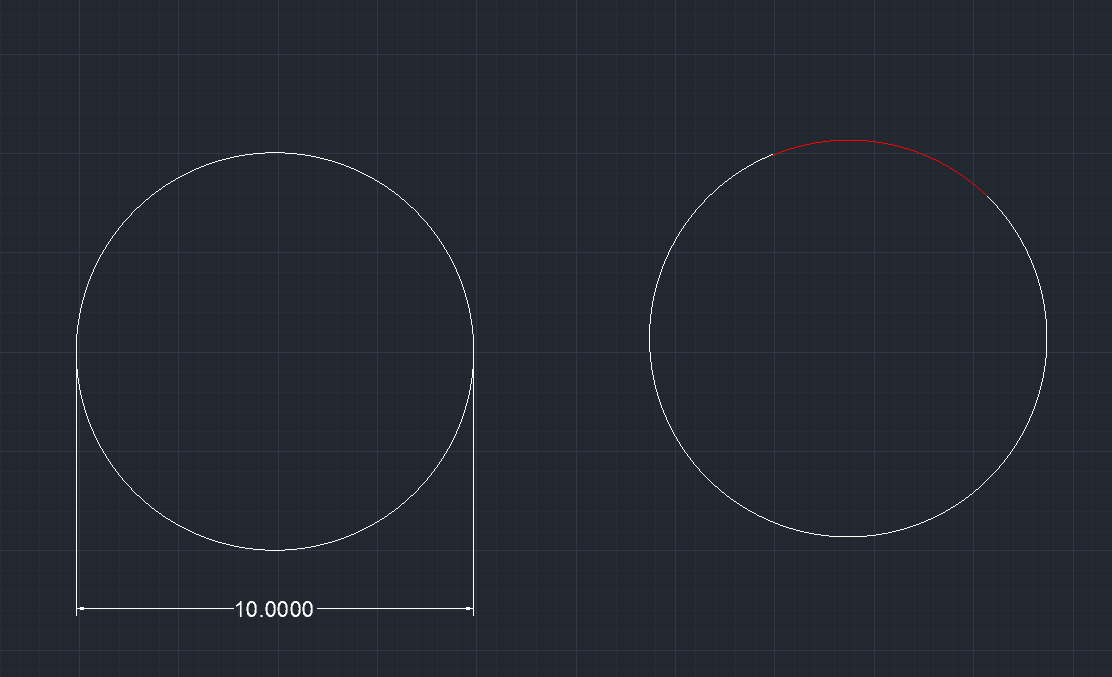# Finding the Center of a Circle

• MHB
• OSUstudent
Once you have them, draw a line between the two points and bisect it with another line. Do this again for a second pair of points. The intersection of these two bisectors will give you the center of the circle. Alternatively, you can use a formula to calculate the center based on the known diameter and a single point within the red area.

#### OSUstudent

Hello!

I have an application where I need to find the center of a circle where I am having trouble coming up with a simple way to do this. The diameter of the circle is known and i want to be able to determine the location of it where only a portion of the circle is known. (see the image below) I will know the location of a single point anywhere in the red circumference could anyone help me with this?Draw the straight line between two points on the circle. Draw the line bisecting that straight line. Do the same with a second pair of points. The two bisectors intersect at the center of the circle.

Thank you for your reply! I am looking for a formula that i could use to calculate where the center of the circle is based on the known diameter and a single point that resides in the red area. Sorry i should have meant to say in the original post that i was looking for a simple formula.

Runner74 said:
Thank you for your reply! I am looking for a formula that i could use to calculate where the center of the circle is based on the known diameter and a single point that resides in the red area. Sorry i should have meant to say in the original post that i was looking for a simple formula.

You're going to need at least two distinct points on the red arc of the circle to find its center.